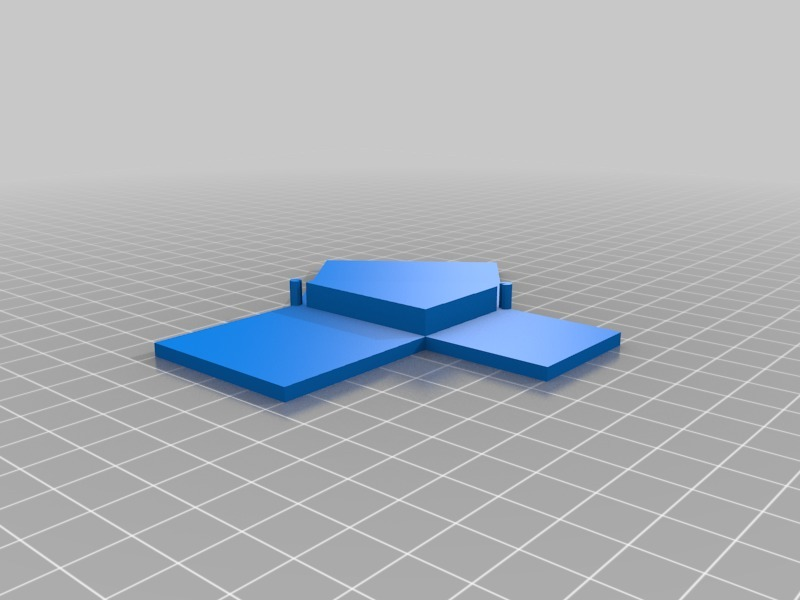# Pythagorean proof

### thingiverse

Pythagorean proof The Pythagorean theorem was known long before Pythagoras, but he may well have been the first to prove it. In any event, the proof attributed to him is very simple, and is called a proof by rearrangement. The two large squares shown in the figure each contain four identical triangles, and the only difference between the two large squares is that the triangles are arranged differently. Therefore, the white space within each of the two large squares must have equal area. Equating the area of the white space yields the Pythagorean theorem, Q.E.D. That Pythagoras originated this very simple proof is sometimes inferred from the writings of the later Greek philosopher and mathematician Proclus.Several other proofs of this theorem are described below, but this is known as the Pythagorean one.

With this file you will be able to print Pythagorean proof with your 3D printer. Click on the button and save the file on your computer to work, edit or customize your design. You can also find more 3D designs for printers on Pythagorean proof.